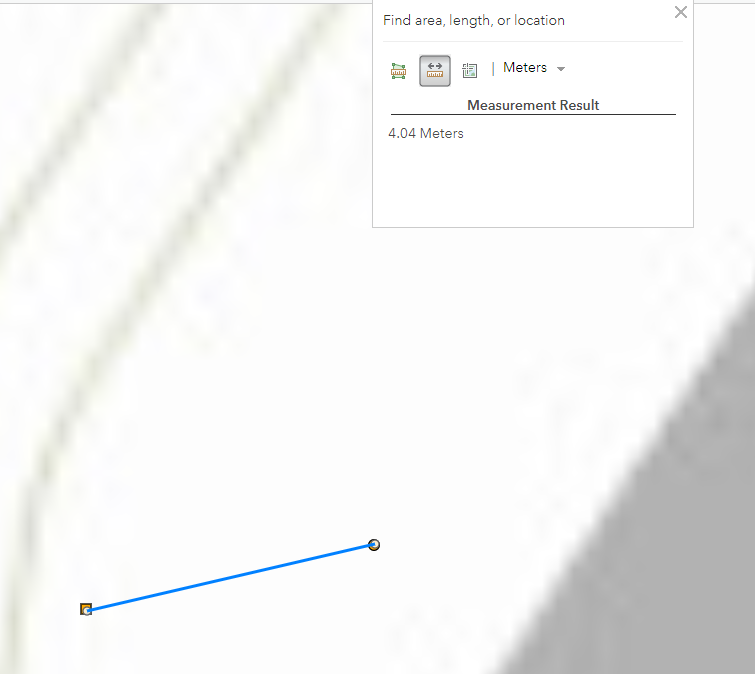# What's the Proper Equation/Code to Get Distances Via Coordinates in Arcade Code

719
7
04-08-2019 08:25 AMNew Contributor III

Using Arcade Code, I've obtained the coordinates between two points and used Vincenty's Formula to calculate the distance between the two points; however, it's not as accurate as the measure tool in the Web Map.

I was wondering what the correct formula I should use for calculating the distance between two points using their coordinates.(I know it depends on the coordinate system, but I thought WGS 1984 would be Vincenty's Formula.)

I also don't want the Planar Distance... so no Distance(Feature1,Feature2) please.

Vincenty's
function Vincenty(lat1,lon1,lat2,lon2){
var x = PI / 180;
var latone = lat1 * x;
var lonone = lon1 * x;
var lattwo = lat2 * x;
var lontwo = lon2 * x;
var deistance = 2 * asin(sqrt(pow(sin((lat1-lat2)/2),2) + cos(lat1) * cos(lat2) * pow(sin((lon1 - lon2) / 2), 2)));
return deistance * 6378137;
}
How I Get the Coordinates
var xthis = Round(Geometry(\$feature).X,2);
var ythis = Round(Geometry
Tags (2)
7 RepliesbyEsri Esteemed Contributor

Normally when you extract the coordinates in ArcGIS Online,they will be returned as Web Mercator Auxiliary Sphere. To get WGS 1984 coordinates you could convert them, however this conversion might not be precise enough for you:

``function MetersToLatLon(x, y) {    // Converts XY point from Spherical Mercator EPSG:900913 to lat/lon in WGS84 Datum    // Fuente: http://www.maptiler.org/google-maps-coordinates-tile-bounds-projection/    var originShift = 2.0 * PI * 6378137.0 / 2.0;    var lon = (x / originShift) * 180.0;    var lat = (y / originShift) * 180.0;    lat = 180.0 / PI * (2.0 * Atan( Exp( lat * PI / 180.0)) - PI / 2.0);    return [lat, lon];}‍‍‍‍‍‍‍‍‍‍‍``

How big is the difference between the distance calculated in Arcade and provided in the Web Map. At what scale did you get these results?New Contributor III

Hey Xander,

I have a result where it's zoomed in all the way.

Measured Distance  = 4.04 MetersArcade Code Vincenty Formula (Also just used the MetersToLatLon): 166.62 MetersbyEsri Esteemed Contributor

That is a huge difference! I just noticed in your code that you round the coordinates with two decimals. I assume that those coordinates are not decimal degrees, since that would cause large errors.

How do you get the "other" geometry? Do you use a FeatureSetBy function for this or do you define the point geometry using json in the expression? What coordinates does each point have?New Contributor III

I get the other geometry by using FeatureSetBy,

The Away is the other geometry coordinates:Do you need my workflow?byEsri Esteemed Contributor

It would be nice to know what your workflow is, but I just did a little test:

``Function Vincenty(lat1, lon1, lat2, lon2) {    var x = PI / 180.0;    var latone = lat1 * x;    var lonone = lon1 * x;    var lattwo = lat2 * x;    var lontwo = lon2 * x;    var dist = 2.0 * Asin(Sqrt(Pow(Sin((lat1-lat2)/2.0), 2.0) + Cos(lat1) * Cos(lat2) * Pow(Sin((lon1 - lon2) / 2.0), 2.0)));    return dist * 6378137.0;}var lat1 = 42.953736914823466;var lon1 = -80.05933367671577;var lat2 = 42.953744767302474;var lon2 = -80.0592853969535;var dist = Vincenty(lat1, lon1, lat2, lon2);Console(dist)// return dist;// 166.6173571575417Function LatLonToMeters(lat, lon) {    // convert from long/lat to google mercator (or EPSG:4326 to EPSG:900913)    // Source: https://gist.github.com/springmeyer/871897    var x = lon * 20037508.34 / 180;    var y = Log(Tan((90 + lat) * PI / 360)) / (PI / 180);    y = y * 20037508.34 / 180;    return [x, y];}// var pnt1 = Point({'x' : lon1, 'y' : lat1, 'spatialReference' : {'wkid' : 3857}});// var pnt2 = Point({'x' : lon2, 'y' : lat2, 'spatialReference' : {'wkid' : 3857}});var xy1 = LatLonToMeters(lat1, lon1);var xy2 = LatLonToMeters(lat2, lon2);var pnt1 = Point({'x' : xy1, 'y' : xy1, 'spatialReference' : {'wkid' : 3857}});var pnt2 = Point({'x' : xy2, 'y' : xy2, 'spatialReference' : {'wkid' : 3857}});var dist2 = Distance(pnt1, pnt2, "meters");Console(dist2);//5.5055826563587return dist2;‍‍‍‍‍‍‍‍‍‍‍‍‍‍‍‍‍‍‍‍‍‍‍‍‍‍‍‍‍‍‍‍‍‍‍‍‍‍‍‍‍‍``

So, the first part does the vicenty calculation and the result is: 166.6173571575417

The second part, converts the lat lon coordinates to web mercator and uses the distance and yields 5.05558265633587 m, which is closer to the 4.04 m in the web map.New Contributor III

I'm guessing the Vincenty code might be wrong or it is not proper for the coordinate system then.

The Distance(pnt1,pnt2,"meters") gives the planar distance, so doesn't account for earth's curvature.byEsri Esteemed Contributor

However, if the distance between the two points is 4 - 5 meters, there is not much curvature you have to account for.